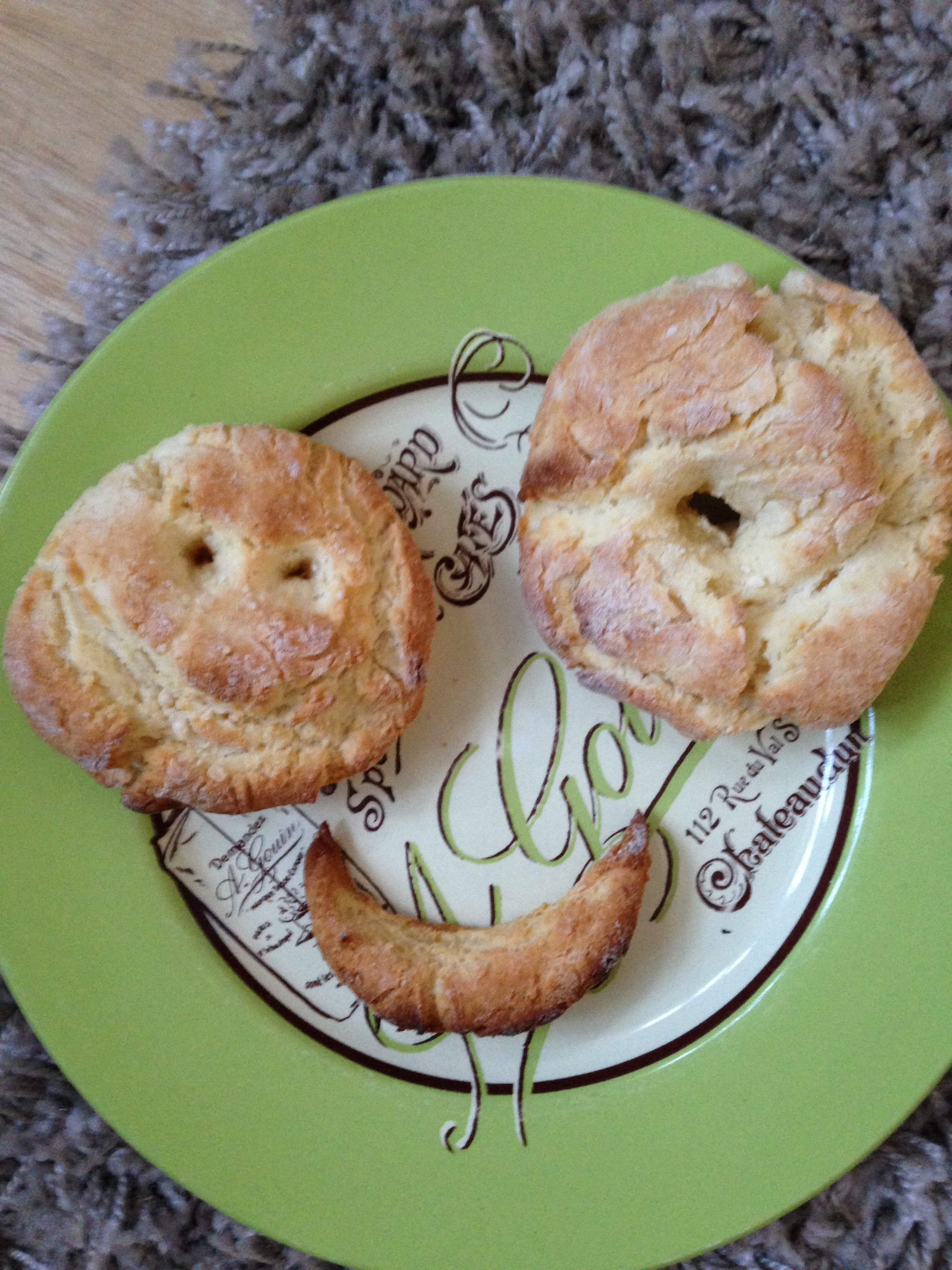# Alan Lauder's Web PageI am a university lecturer in the Mathematical Institute, with the title of Professor of Mathematics, and a tutorial fellow in mathematics at Hertford College.

My interests are in computational number theory, p-adic cohomology, elliptic curves and modular forms. I can be contacted at "lauder" at "maths.ox.ac.uk".

I am an executive editor of the Quarterly Journal of Mathematics.

I co-organised a conference on Effective methods in p-adic cohomology and also a conference on Rational points on curves.

## Recent Papers:

• (with Henri Darmon) Stark points on elliptic curves via Perrin-Riou's philosophy, Annales Mathématiques du Québec, to appear. PDF
• (with Jan Vonk) Computing p-adic L-functions of totally real fields, Mathematics of Computation 91 (2022), 921-942. PDF
• (with Henri Darmon and Victor Rotger) First order p-adic deformations of weight one newforms, in "Heidelberg conference on L-functions and automorphic forms", Bruinier and Kohnen (eds), Springer, 39-80, 2017. PDF
• (with Kevin Buzzard) A computation of modular forms of weight one and small level PDF. There is an accompanying webpage containing data on weight one modular forms. Annales Mathématiques du Québec, Volume 41, Issue 2, (2017), 213-219.
• (with Henri Darmon and Victor Rotger) Overconvergent generalised eigenforms of weight one and class fields of real quadratic fields, Advances in Mathematics 283 (2015), 130-142. PDF (Erratum: the field on the second line of Example 1.1 should be K(√(3 + √21)/2).)
• (with Henri Darmon and Victor Rotger) Gross-Stark units and p-adic iterated integrals attached to modular forms of weight one, Annales Mathématiques du Québec, Volume 40, Issue 2, (2016), 325 - 354. PDF
• (with Henri Darmon and Victor Rotger) Stark points and p-adic iterated integrals attached to modular forms of weight one, Forum of Mathematics, Pi (2015), Vol. 3, e8, 95 pages. PDF Journal Version (There are two typographical errors in the table in Appendix A, the levels in Examples 3.14 and 3.15 being 69 and 161 and not as stated 57 and 35, respectively.)
• Efficient computation of Rankin p-adic L-functions, in "Computations with Modular Forms, Proceedings of a Summer School and Conference, Heidelberg, August/September 2011", Boeckle G. and Wiese G. (eds), Springer Verlag, 181-200, 2014. PDF
• Computations with classical and p-adic modular forms, LMS J. Comput. Math. 14, (2011), 214-231 PDF. (Freely available via JCM website.) Code for Algorithms 1 and 2 in SAGE: Alg1and2.sage. A fully documented python version of this sage file: Alg1and2.py. Code for Algorithms 1 and 2 in MAGMA: Alg1and2.m. (The algorithms are now included in the distributed versions of MAGMA and SAGE.)## Older Publications:

• Degenerations and limit Frobenius structures in rigid cohomology, LMS J. Comp. Math. Vol. 14, 1-33, 2011. Freely available via JCM website. PDF
• Ranks of elliptic curves over function fields. LMS J. Comp. Math. Vol. 11, 172-212, 2008. Freely available at JCM website. PDF (Erratum: the deduction in the first sentence of the proof of Corollary 3.5 is incorrect. A more refined argument is required, taking into account the steps in the filtration, and so the exponent in the power of p in the statement should be multiplied by 2. The electronic appendices are no longer on the journal website, so I have put them here: d6 d12 d18 d24 d30 RI )
• A recursive method for computing zeta functions of varieties, LMS J. Comp. Math. Vol. 9, 222-267, 2006. Freely available at JCM website. PDF
• (with Fatima Abu Salem and Shuhong Gao) Factoring polynomials via polytopes, Proceedings of ISSAC (International Symposium on Symbolic and Algebraic Computation) 2004, Gutierrez (Ed), 4-11. PDF
• Counting solutions to equations in many variables over finite fields, Foundations of Computational Mathematics Vol. 4 No. 3, (2004), 221-267. PDF
• Deformation theory and the computation of zeta functions, Proceedings of the London Mathematical Society, Vol. 88 Part 3, (2004), 565-602. PDF
• (with Daqing Wan) Computing zeta functions of Artin-Schreier curves over finite fields II, Journal of Complexity, Vol. 20 (2004), 331-349. PDF
• Computing zeta functions of Kummer curves via multiplicative characters, Foundations of Computational Mathematics, Vol 3 No. 3, pages 273-295, 2003. PDF
• Zero-patterns of polynomials and convex polytopes, J. of Combinatorial Theory Series A, Vol. 102 (No.1), (2003), 10-15. PDF
• (with Daqing Wan) Computing zeta functions of Artin-Schreier curves over finite fields, London Mathematical Society JCM Volume 5, (2002), pp 34-55. PDF. Freely available at JCM website.
• (with Richard Brent and Shuhong Gao) Random Krylov spaces over finite fields, SIAM J. on Discrete Mathematics, Vol. 16 No. 2, (2003), 276-287. PDF
• (with Daqing Wan) Counting points on varieties over finite fields of small characteristic, in "Algorithmic Number Theory: Lattices, Number Fields, Curves and Cryptography" (Mathematical Sciences Research Institute Publications), J. P. Buhler and P. Stevenhagen (eds.), Cambridge University Press, 579-612, 2008. (Version Oct 2002) PDF
• (with Shuhong Gao and Erich Kaltofen) Deterministic distinct-degree factorisation of polynomials over finite fields, Journal of Symbolic Computation, Vol. 38 No. 6, (2004), 1461-1470. PDF
• (with Kenny Paterson) Computing the error linear complexity spectrum of a binary sequence of period 2^n, IEEE Trans. Info. Theory Vol 49 No 1, (2003), 273-280. PDF
• (with Shuhong Gao) Hensel lifting and bivariate polynomial factorisation over finite fields, Math. Comp. 71, No. 240, (2002), 1663-1676. PDF
• (with Shuhong Gao) Decomposition of polytopes and polynomials, Discrete Comput. Geom 26, 89-104 (2001) PDF
• Continued fractions of Laurent series with partial quotients from a given set, Acta Arithmetica XC.3, 252-271 (1999) PDF
• Polynomials with odd orthogonal multiplicity, Finite Fields and Their Applications 4, 453-464 (1998) PDF
• Continued fractions and sequences, Ph.D. London University 1999 PDF

## Expository Papers:

• Rationality and meromorphy of zeta functions, Finite Fields and Their Applications 11 (2005), 491-510. PDF
• Rigid cohomology and p-adic point counting, J. Theor. Nombres Bordeaux 17 (2005), no. 1, 169-180. PDF
• Homotopy methods for equations over finite fields, in "Applied Algebra, Algebraic Algorithms and Error-Correcting Codes", Fossorier, Hoholdt and Poli (eds), LNCS 2643,18-24, 2003. PDF